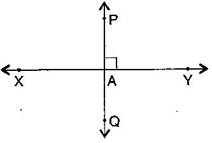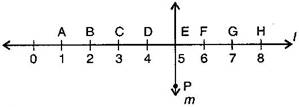### Understanding Elementary Shapes-Solutions Ex-5.5

CBSE Class –VI Mathematics
NCERT Solutions
Chapter 5 Understanding Elementary Shapes (Ex. 5.5)

Question 1. Which of the following are models for perpendicular lines:
(a)The adjacent edges of a table top.
(b)The lines of a railway track.
(c)The line segments forming the letter ‘L’.
(d)The letter V.
(b) Not perpendicular, they are parallel
(c) Perpendicular
(d) Not perpendicular
Question 2. Let $\overline{\text{PQ}}$ be the perpendicular to the line segment $\overline{\text{XY}}.$ Let $\overline{\text{PQ}}$ and $\overline{\text{XY}}$ intersect in the point A. What is the measure of $\mathrm{\angle }$PAY.$\mathrm{\angle }$PAY = ${90}^{\circ }$
Question 3. There are two “set-squares” in your box. What are the measures of the angles that are formed at their corners? Do they have any angle measure that is common?
Answer: One set-square has 45o,90o,45o  and other set-square has 60o,90o,30o . They have 90o as common angle.
Question 4. Study the diagram. The line $l$ is perpendicular to line $m.$(a) Is CE = EG?
(b)   Does PE bisect CG?
(c) Identify any two line segments for which PE is the perpendicular bisector.
(d) Are these true?
(i) AC > FG
(ii) CD = GH
(iii) BC < EH
Answer: (a) Yes, both measure 2 units.
(b) Yes, because CE = EG
(c) $\overline{\text{DF}}$ and $\overline{\text{CG}}$$\overline{\text{BH}}$
(d) (i) True, (ii) True, (iii) True